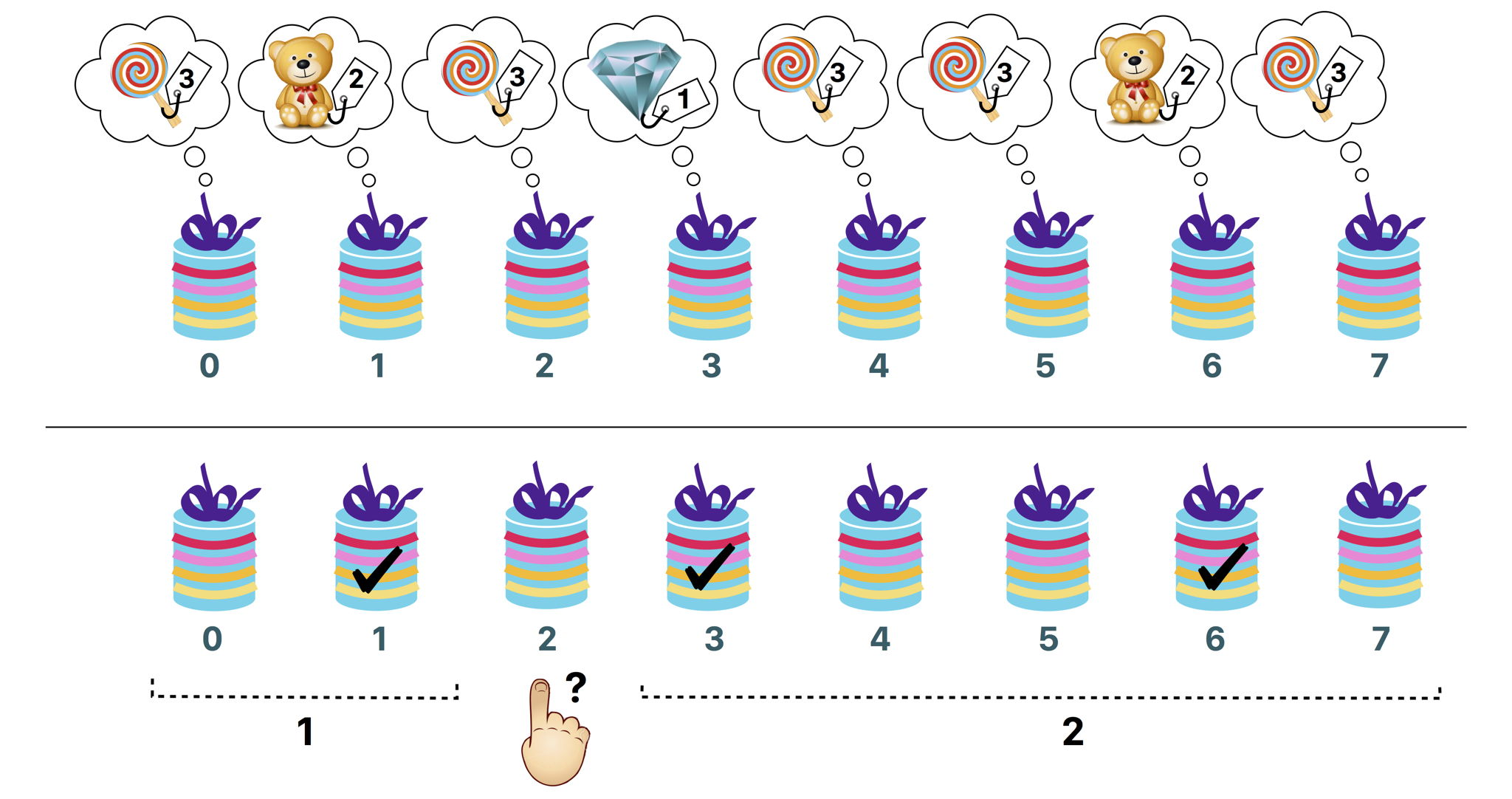시간 제한메모리 제한제출정답맞힌 사람정답 비율
1 초 1024 MB64181634.783%

## 문제

The Big Prize is a famous TV game show. You are the lucky contestant who has advanced to the final round. You are standing in front of a row of $n$ boxes, labeled $0$ through $n-1$ from left to right. Each box contains a prize that cannot be seen until the box is opened. There are $v \geq 2$ different types of prizes. The types are numbered from $1$ to $v$ in decreasing order of value.

The prize of type $1$ is the most expensive one: a diamond. There is exactly one diamond in the boxes. The prize of type $v$ is the cheapest one: a lollipop. To make the game more exciting, the number of cheaper prizes is much larger than the number of more expensive ones. More specifically, for all $t$ such that $2 \leq t \leq v$ we know the following: if there are $k$ prizes of type $t-1$, there are strictly more than $k^2$ prizes of type $t$.

Your goal is to win the diamond. At the end of the game you will have to open a box and you will receive the prize it contains. Before having to choose the box to open you get to ask Rambod, the host of the game show, some questions. For each question, you choose some box $i$. As his answer, Rambod will give you an array $a$ containing two integers. Their meaning is as follows:

• Among the boxes to the left of box $i$ there are exactly $a$ boxes that contain a more expensive prize than the one in box $i$.
• Among the boxes to the right of box $i$ there are exactly $a$ boxes that contain a more expensive prize than the one in box $i$.

For instance, suppose that $n=8$. For your question, you choose the box $i=2$. As his response, Rambod tells you that $a=[1,2]$. The meaning of this response is:

• Exactly one of the boxes $0$ and $1$ contains a prize more expensive than the one in box $2$.
• Exactly two of the boxes $3, 4, \ldots, 7$ contain a prize more expensive than the one in box $2$.

Your task is to find the box containing the diamond by asking a small number of questions.

## 구현

You should implement the following procedure:

int find_best(int n)

• This procedure is called exactly once by the grader.
• $n$: the number of boxes.
• The procedure should return the label of the box which contains the diamond, i.e., the unique integer $d$ ($0 \leq d \leq n-1$) such that box $d$ contains a prize of type $1$.

The above procedure can make calls to the following procedure:

int[] ask(int i)

• $i$: label of the box that you choose to ask about. The value of $i$ must be between $0$ and $n-1$, inclusive.
• This procedure returns the array $a$ with $2$ elements. Here, $a$ is the number of more expensive prizes in the boxes to the left of box $i$ and $a$ is the number of more expensive prizes in the boxes to the right of box $i$.

## 예시

The grader makes the following procedure call:

find_best(8)


There are $n=8$ boxes. Suppose the prize types are $[3,2,3,1,3,3,2,3]$. All possible calls to the procedure ask and the corresponding return values are listed below.

• ask(0) returns $[0, 3]$
• ask(1) returns $[0, 1]$
• ask(2) returns $[1, 2]$
• ask(3) returns $[0, 0]$
• ask(4) returns $[2, 1]$
• ask(5) returns $[2, 1]$
• ask(6) returns $[1, 0]$
• ask(7) returns $[3, 0]$

In this example, the diamond is in box $3$. So the procedure find_best should return $3$.The above figure illustrates this example. The upper part shows the types of the prizes in each box. The lower part illustrates the query ask(2). The marked boxes contain more expensive prizes than the one in box $2$.

## 제한

• $3 \leq n \leq 200\,000$.
• The type of the prize in each box is between $1$ and $v$, inclusive.
• There is exactly one prize of type $1$.
• For all $2 \leq t \leq v$, if there are $k$ prizes of type $t-1$, there are strictly more than $k^2$ prizes of type $t$.

## 서브태스크

번호 배점 제한
1 20

There is exactly $1$ diamond and $n-1$ lollipops (hence, $v = 2$). You can call the procedure ask at most $10\,000$ times.

2 80

## 점수

In subtask 2 you can obtain a partial score. Let $q$ be the maximum number of calls to the procedure ask among all test cases in this subtask. Then, your score for this subtask is calculated according to the following table:

Questions Score
$10\,000 < q$ $0$ ('Wrong Answer')
$6000 < q \leq 10\,000$ $70$
$5000 < q \leq 6000$ $80 - (q-5000)/100$
$q \leq 5000$ $80$

## 샘플 그레이더

The sample grader is not adaptive. Instead, it just reads and uses a fixed array $p$ of prize types. For all $0 \leq b \leq n-1$, the type of the prize in box $b$ is given as $p[b]$. The sample grader expects input in the following format:

• line $1$: $\;\; n$
• line $2$: $\;\; p \;\; p \; \ldots \; p[n-1]$

The sample grader prints a single line containing the return value of find_best and the number of calls to the procedure ask.

## 출처• 문제를 만든 사람: Hamed Valizadeh

## 제출할 수 있는 언어

C++17, C++14, C++20, C++14 (Clang), C++17 (Clang), C++20 (Clang)

## 채점 및 기타 정보

• 예제는 채점하지 않는다.